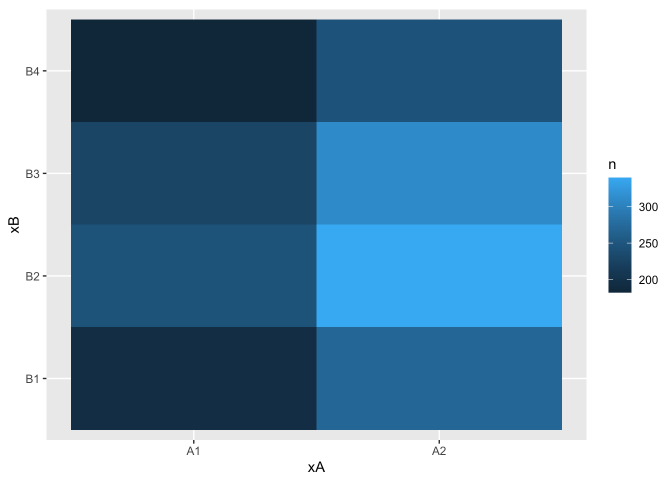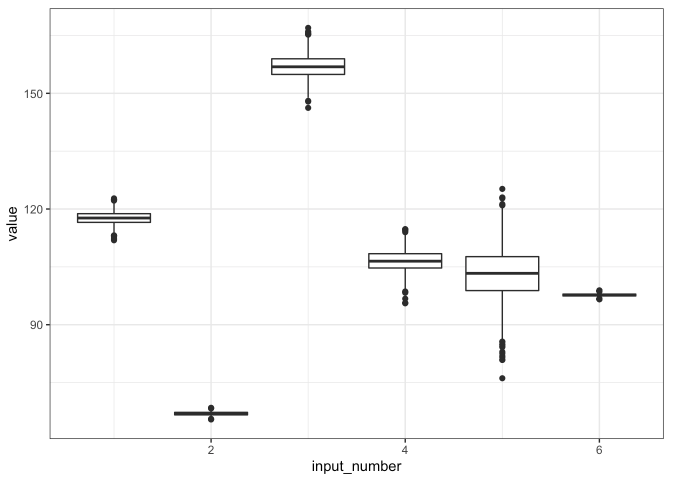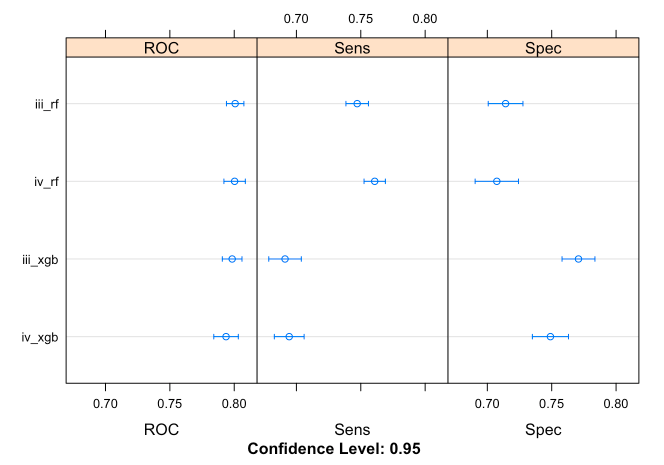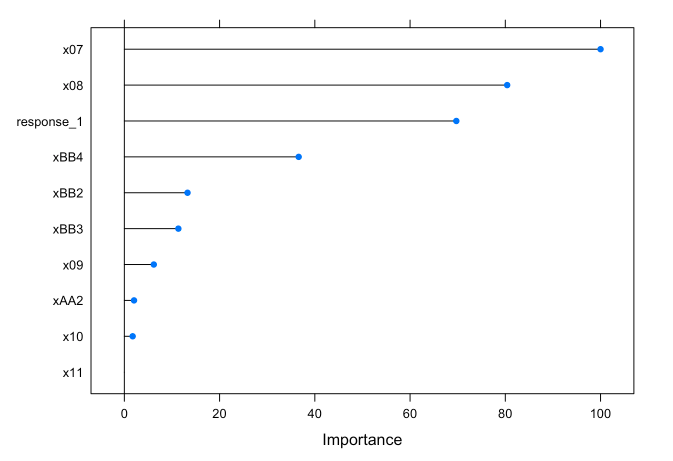< home

# Machine Learning Methods in R

An almost comprehensive project comparing and analyzing basic machine learning methods, from simple linear regression to support vector machines and random forest, on a moderate-size construction raw materials dataset with 12 continuous inputs and two discrete inputs.

## Machine Learning Methods Used

• Simple Linear Regression
• with basis functions
• Regularized Regression with Elastic net
• pair and triplet interactions
• Logistic Regression
• Generalized Linear Regression
• Neural Network
• single hidden layer, regression and classification
• Random Forest
• regression and classification
• regression and classification
• Support Vector Machines
• regression and classification
• Multivariate Additive Regression Splines (MARS)
• regression and classification
• Partial Least Squares

## Code

Exploratory Data Analysis (EDA):

# Some code snippets

data_url <- 'https://raw.githubusercontent.com/jyurko/CS_1675_Fall_2020/master/HW/final_project/cs_1675_final_project_data.csv'

step_1_df <- df %>% select(xA, xB, x01:x06, response_1)

# Variation within variables
## Boxplots
step_1_df %>% tibble::rowid_to_column() %>%
tidyr::gather(key = "key", value = "value", -rowid, -response_1, -xA, -xB) %>%
mutate(input_number = as.numeric(stringr::str_extract(key, "\\d+"))) %>%
ggplot(mapping = aes(x = input_number, y = value)) +
geom_boxplot(mapping = aes(group = input_number)) +
theme_bw()# Covariation between variables
## geom_tile (between categorical inputs)
df %>%
count(xA, xB) %>%
ggplot(mapping = aes(x = xA, y = xB)) +
geom_tile(mapping = aes(fill = n))Examine part i

Regression Analysis:

# lm() to all inputs
mod_lm_step1 <- lm(response_1 ~ ., step_1_df)

# lm() to a self-devised basis function
mod_lm_basis <- lm(response_1 ~ xA + xB*(splines::ns(x01, df = 2) + splines::ns(x02, df = 2) + splines::ns(x03, df = 2)), step_1_df)


Binary Classification Analysis:

# Training control settings
my_ctrl <- caret::trainControl(method = "repeatedcv",
number = 5,
repeats = 5,
savePredictions = TRUE,
summaryFunction = twoClassSummary,
classProbs = TRUE)
roc_metric <- "ROC"

# Logistic regression with additive terms
set.seed(12345)
mod_glm <- caret::train(outcome_2 ~ .,
method = "glm",
metric = roc_metric,
trControl = my_ctrl,
preProcess = c("center", "scale"),
data = step_2_b_df)

confusionMatrix.train(mod_glm)

# Regularized regression with elastic net
## Custom tuning grid
enet_grid <- expand.grid(alpha = seq(0.1, 0.9, by = 0.1),
lambda = exp(seq(-6, 0.5, length.out = 25)))

set.seed(12345)
mod_glmnet_2_b <- caret::train(outcome_2 ~ (.)^2,
method = "glmnet",
preProcess = c("center", "scale"),
tuneGrid = enet_grid,
metric = roc_metric,
trControl = my_ctrl,
data = step_2_b_df)


Interpretation of Results:

# Evaluating response_1 as a feature
## Area under the ROC curve (AUC)
results <- resamples(list(iii_rf = iii_models$iii_rf, iii_xgb = iii_models$iii_xgb,
iv_rf = iv_models$iv_rf, iv_xgb = iv_models$iv_xgb))

dotplot(results)

## Based on AUC, models including response_1 (intermediate input) as feature yield better performance in predicting outcome_2 (output variable); observed from the higher AUC values# Variable importance
## Models with response_1 as a feature
plot(varImp(iii_models\$iii_rf))Examine part v